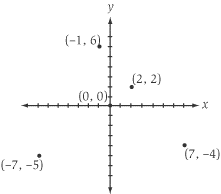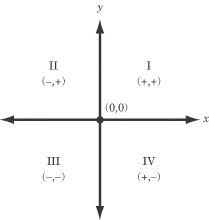SAT数学分析：The Coordinate Plane

来源：新东方在线    要点：SAT数学分析The coordinate plane is a plane determined by two perpendicular lines, the x-axis and the y-axis. The x-axis is the horizontal axis, and the y-axis is the vertical axis. Every point in the plane can be stated by a pair of coordinates that express the location of the point in terms of the two axes. The intersection of the x- and y-axes is designated as the origin, and its point is (0, 0).As you can see from the figure, each of the points on the coordinate plane is expressed by a pair of coordinates: (x, y). The first coordinate in a coordinate pair is called the x-coordinate. The x-coordinate is the point’s location along the x-axis and can be determined by the point’s distance from the y-axis (where x = 0). If the point is to the right of the y-axis, its x-coordinate is positive, and if the point is to the left of the y-axis, its x-coordinate is negative. The second coordinate in a coordinate pair is the y-coordinate. The y-coordinate of a point is its location along the y-axis and can be calculated as the distance from that point to the x-axis. If the point is above the x-axis, its y-coordinate is positive, and if the point is below the x-axis, its y-coordinate is negative.The Quadrants The coordinate plane is divided into four quadrants. Each quadrant is a specific region in the coordinate plane. The region in which x > 0 and y > 0 is Quadrant I. The region in which x < 0 and y > 0 is Quadrant II. The region in which x < 0 and y < 0 is Quadrant III. The region in which x > 0 and y < 0 is Quadrant IV.For example, the point (4, –2) lies in quadrant IV, with an x-coordinate that is 4 units to the right of the y-axis and a y-coordinate that is 2 units below the x-axis. This is how the coordinates of a point specify its exact location. The coordinates of the origin are, by definition, (0, 0).